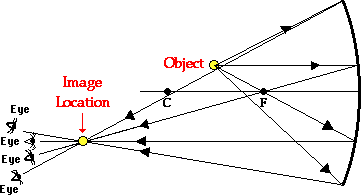Home » Posts tagged 'Optics' (Page 2)

# Tag Archives: Optics

## Image formed by a thick Plane Mirror

“A thick plane mirror forms a number of images of a point source of light . which image is brightest ?”

Answer: In the case of a thick plane mirror, reflection takes place at the twosurfaces, (top and bottom surfaces) and images are formed due to both. The second surface is silvered and therefore the second image is the brightest. Further images are formed due to multiple reflections and due to absorption of light by medium the images become fainter.

If the front surface of the mirror is silvered, only one image will be formed.

## Optics MCQ – image formation by concave mirror

I am having trouble with a problem.

Q. In an experiment to determine the focal length (f) of a concave mirror by the u–v method, a student places the object pin A on the principal axis at a distance x from the pole P. The student looks at the pin and its inverted image from a
distance keeping his/her eye in line with PA. When the student shifts his/her eye towards left, the image appears to the right of the object pin. Then

(A) x < f

(B) f < x < 2f

(C) x = 2f

(D) x > 2fEXPLANATION

The image and the object will move together at 2f

The one closer to the eye will move faster

In the question, the situation occurs when the image is closer to the eye and this happens when the object is between f and 2f

## Five Sure Shot portions for SA2 (Class X CBSE)

The students appearing for SA2 of CBSE class X are requested to practice the following portions of Physics very well so that they can get excellently good marks in Science.

1. Practice all ray diagrams showing image formation by concave mirror, convex mirror, convex lens and concave lens along with characteristics of image.
2. Defects of eye, cause and rectification
3. Structure of human eye (diagram), function of each part, power of accommodation and persistence of vision.
4. Refraction through a glass slab and a glass prism, lateral displacement and angle of deviation.
5. Scattering of light, why sky is blue, Tyndal effect.
6. Also Practice numerical based on mirror formula, lens formula, refractive index.

## A numerical Problem from optics

When an object is kept at a distance of 60cm from a concave mirror, the magnification is 1/2. Where should the object be placed to get magnification of 1/3??

Ans:

In the first part, u=-60, m=(-)1/2 implies v=-30 cm (Since the image must be real as concave lens form virtual image of bigger size only)

we get f = –20 cm

In II case

m=-1/3

v=u/3

f=-20cm

we get u=4f=4 x (-20)=-80 cm

## Propagation of Light, Group velocity and Phase velocity

“how can light travel in the form of waves in space, which has no medium? if light doesn’t travel in the form of wave in the space then in equation. ‘phase velocity=c^2/group velocity ‘ phase velocity becomes zero and also c=0, which indicates velocity of light =0, but light actually travel in space . How can this be possible?”

I feel that there is some sort of misunderstanding in your concept.

Why should phase velocity become zero?

Please remember that light is an electromagnetic wave, where the propagation is due to the mutually reinforcing time varying electric and magnetic field vectors varying in mutually perpendicular directions.

The phase velocity is a the speed at which a point of fixed phase (say the crust of the wave)  propagates, which is not always the speed at which the electromagnetic information travels.

Group velocity is the speed at which electromagnetic information travels.

## Transverse nature of light

it is said that in tranverse waves the particles move perpendicular to the direction of motion of wave.
it is also said that light is a transverse wave.
but how can the particles in light move at all, as light doesn’t require a medium to travel?

Light is an electromagnetic wave, energy propagated as a combination of electric and magnetic fields varying with time in mutually perpendicular directions, also perpendicular to the direction of propagation. This makes light a transverse wave.

## Why does a test tube seem silvery when put in water?

Why does a test tube seem silvery when put in water?The air bubbles trapped in a glass paper weight a;lso shines like silver due to TIR.